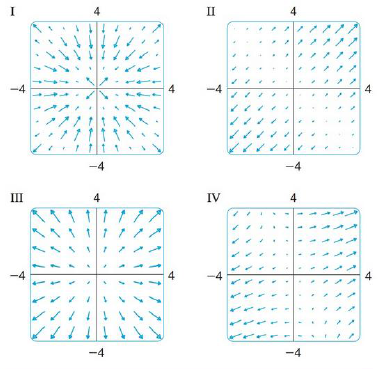Chapter 16.1, Problem 31E

Chapter
Section
Textbook Problem

Match the functions f with the plots of their gradient vector fields labeled I-IV. Give reasons for your choices.31. f(x, y) = (x + y)2To determine

To match: The function f(x,y)=(x+y)2 with the plots labeled as I-IV.

Explanation

Given data:

f(x,y)=(x+y)2 (1)

Formula used:

Write the expression for gradient vector field of a two dimensional vector.

f(x,y)=fxi+fyj (2)

Write the required differentiation formulae with respect to x as follows.

x(x2)=2xx(2xy)=2yx(y2)=0

Write the required differentiation formulae with respect to y as follows.

y(y2)=2yy(2xy)=2xy(x2)=0

Rearrange equation (1) as follows.

f(x,y)=(x+y)2=x2+y2+2xy

Differentiate the term x2+y2+2xy with respect to x .

x(x2+y2+2xy)=x(x2)+x(y2)+x(2xy)=2x+0+2y=2x+2y=2(x+y)

Differentiate the term x2+y2+2xy with respect to y

Still sussing out bartleby?

Check out a sample textbook solution.

See a sample solution

The Solution to Your Study Problems

Bartleby provides explanations to thousands of textbook problems written by our experts, many with advanced degrees!

Get Started

Evaluate the expression sin Exercises 116. (23)2

Finite Mathematics and Applied Calculus (MindTap Course List)

A population of N = 7 scores has a mean of = 13. What is the value of X for this population?

Essentials of Statistics for The Behavioral Sciences (MindTap Course List)

In Exercises 7-28, perform the indicated operations and simplify each expression. 16. x1x+2x+3x21

Applied Calculus for the Managerial, Life, and Social Sciences: A Brief Approach

In problems 59 and 60, simplify each expression. 59.

Mathematical Applications for the Management, Life, and Social Sciences

Divide the following fractions and reduce to lowest terms. 28.

Contemporary Mathematics for Business & Consumers

ln |2x + 1| + C 2 ln |2x + 1| + C

Study Guide for Stewart's Single Variable Calculus: Early Transcendentals, 8th

The equation of the line through (4, 10, 8) and (3, 5, 1) is:

Study Guide for Stewart's Multivariable Calculus, 8th